# Rendering Twisty Thingies

I’ve got a program called Twist Flux. It features an array of twisty, bendy… thingies.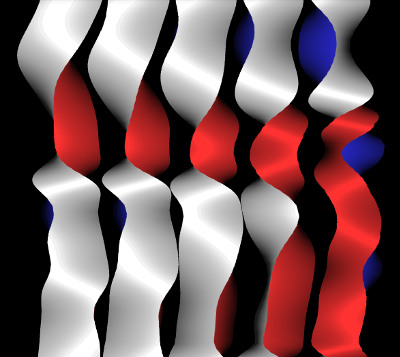I found a way to render twisty shapes which appear to have volume without going so far as to use 3D models of twisted rods.

First, a bit about the underlying simulation. I committed to a very simple 2D model because I wanted to have an array of lots of twisty thingies, and I wanted them to interact and blend their twistinesses with their neighbors, and I wanted to not use all the CPU time in the universe.

I’ll call the thingies “twistors”. Each twistor is made up of a fixed number of points evenly spaced along the vertical. Because their vertical positions are fixed, these points don’t need to carry a y-value. Each point carries two “position” values: horizontal displacement and twist angle (radians). As for rendering, horizontal displacement is straightforward…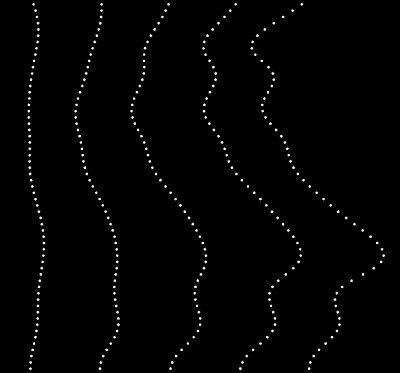… but there are many ways to draw a shape based on that twist value. One of the simplest shapes to draw is an infinitely-thin ribbon. All you need to do is draw a wide line along the points using the cosine of the twist angle to determine the width at each point. That same cosine can be used to do a basic lighting effect: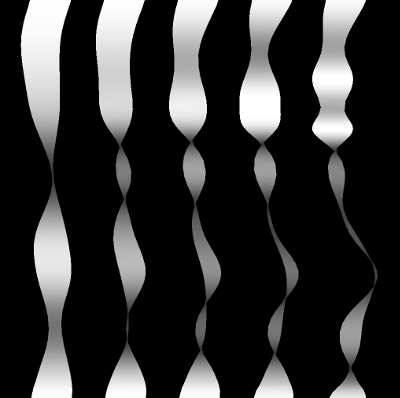That looks kinda twisty. It doesn’t look great, but it is very computationally cheap. A proper twisted shape is more complex.Unlike twisting a ribbon (a shape having a line segment for its cross-section), when you twist something which has a polygon cross-section you will see multiple faces of that polygon at some points. This complicates drawing twistors, especially if you want the different sides to have different colors. You can’t just vary the width of a line, you need to draw more than one color at some points along the twistor.

I thought I was stuck with this complication and that I would have to draw the multiple sides of the twisted shape with multiple varying-width lines. Each of those wide lines would have much more complex offset and width than the simple ribbon case, and I didn’t get too far with the idea. At some point you have to give up on trickery and just model the thing in 3D.

But I didn’t wanna model the thing in 3D.

Turns out there’s a cheap way to draw a fancy twist: create an image of one complete rotation, then sample that texture using twist angle as one of the coordinates. Essentially you stretch the image of a single twist, tending towards infinite stretch where there’s zero change in twist.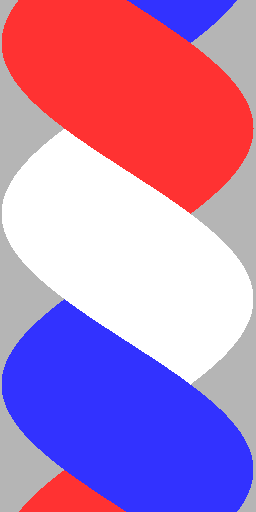``````generateTwist {
bmp = createBitmap(width, height);
aStep = 2 * PI / 3;
for(y = 0; y < height; y++) {
a = (y / height) * (2 * PI);
x1 = width * (0.5 + 0.5 * cos(a));
x2 = width * (0.5 + 0.5 * cos(a + aStep));
x3 = width * (0.5 + 0.5 * cos(a + 2 * aStep));
for(x = x2; x < x3; x++)
bmp.setPixel(x, y, white);
for(x = x3; x < x1; x++)
bmp.setPixel(x, y, red);
for(x = x1; x < x2; x++)
bmp.setPixel(x, y, blue);
}
}``````Now that looks twisty. Fancy lighting can be made just as cheap by generating a normal map with a very similar bit of code: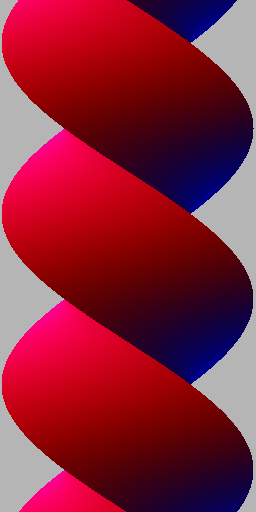The fragment shader making use of those two textures looks like this:

``````uniform sampler2D uColorTexture;
uniform sampler2D uNormalTexture;
uniform vec3 uLight;

varying float vLateral;
varying float vTwist;

vec3 decodeRGBvector(vec3 rgb) {
return (rgb.xyz - 0.5) * 2.0;
}

void main() {
vec2 st = vec2(vLateral, vTwist);
vec4 c = texture2D(uColorTexture, st);
vec3 normal = decodeRGBvector(texture2D(uNormalTexture, st).rgb);
float l = max(0.0, dot(normal, uLight));
gl_FragColor = vec4(c.rgb * (0.1 + 0.7 * l + 0.2 * power(l)), c.a);
}``````

So there you have it, twisty things with apparent volume and shiny lighting, and the cost per pixel is just two texture samples and a dot product.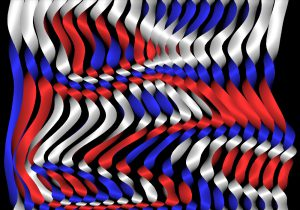This entry was posted in Uncategorized and tagged . Bookmark the permalink.